July 17, 2019

# NP-Completeness

• Computers and Intractability: A Guide to the Theory of NP-Completeness, Michael R. Garey and David S. Johnson, Series of Books in the Mathematical Sciences, W. H. Freeman, 1979.

# Content

• Part 1: Introduction - Computers, Complexity, and Intractability
• Part 2: The Theory of NP-Completeness

# Theory of NP-Completeness

• Provides techniques to prove that a problem is just as hard as a set of other problems that are recognized as being difficult
• Prove that a problem is equivalent to another problem that is hard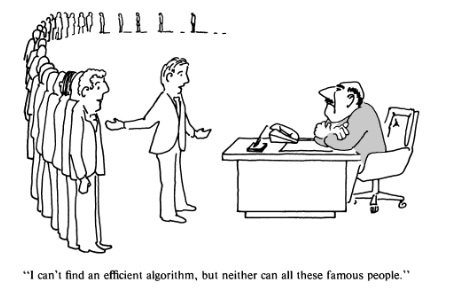# Theory of NP-Completeness

• Finding out that a problem is NP-complete is the first step to work with it
• The problem at hand (i.e. in the industry) still needs a solution
• Discovering a problem is NP-complete provides information
• About the approach(es) we need to follow to solve the problem
• Trying to design an exact algorithm should have a low priority

# Theory of NP-Completeness

• With NP-complete problems, we look for less ambitious approaches
• Concentrate in special cases of the general problem
• Algorithms that do not run quick but find a solution most of the time
• Relax the problem by removing some constraints
• Why We Need NP-completeness Theory
• Assist algorithm designers to focus toward approaches that lead to useful algorithms

# Basic Concepts

• Problem
• A general question to be answered
• Has several parameters or free variables with unspecified values
• Problem description
• A general description of all the problem parameters
• A statement of what properties the answer or solution to the problem must satisfy
• Instance of a problem
• Specification of particular values for all parameters of a problem

# Basic Concepts

• Example of the “traveling salesman problem”
• cities: $$C = \{c_1, c_2, \dots, c_m\}$$
• distances: $$d(c_i, c_j)$$ the distance between each pair of cities
• solution: an ordering $$\langle c_{\pi(1)}, c_{\pi(2)}, \dots, c_{\pi(m)} \rangle$$ of the given cities minimizing
$$\Biggl[\sum\limits_{i=1}^{m-1} d(c_{\pi(i)}, c_{\pi(i+1)}) + d(c_{\pi(m)}, c_{\pi(1)})\Biggr]$$

# Basic Concepts

• Example of the “traveling salesman problem” $$\dots$$
• A tour that starts at the first city, visits each city in sequence, and returns to the first city from the last city
• First city: $$c_{\pi(1)}$$
• Last city: $$c_{\pi(m)}$$
• An instance
• $$C = \{c_1, c_2, c_3, c_4\}$$, $$d(c_1, c_2) = 10$$, $$d(c_1, c_3) = 5$$, $$d(c_1, c_4) = 9$$, $$d(c_2, c_3) = 6$$, $$d(c_2, c_4) = 9$$, $$d(c_3, c_4) = 3$$
• Ordering $$\langle c_1, c_2, c_4, c_3 \rangle$$ is a solution with length 27

# Basic Concepts

• Example of the “traveling salesman problem” $$\dots$$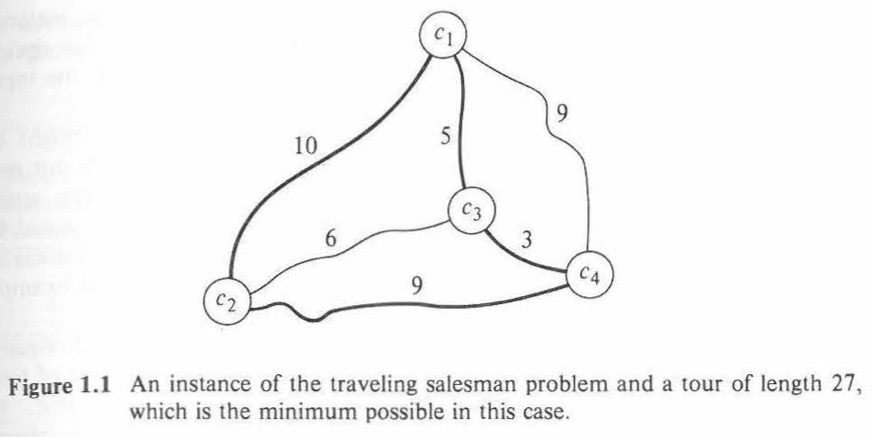# Basic Concepts

• Algorithm
• Step by step procedure to solve problems
• A computer program
• An algorithm solves a problem $$\Pi$$ if that algorithm can be applied to any instance $$I$$ of $$\Pi$$ and is always guaranteed to produce a soluction for that instance $$I$$.
• An algorithm does not solve the traveling salesman problem unless it always constructs an ordering that gives a minimum length tour

# Basic Concepts

• Efficient
• We are interested in finding the most efficient algorithm to solve a problem
• Involves various computing resources needed to execute an algorithm
• We concentrate on time

# Basic Concepts

• Time requirements
• Conveniently expressed in terms of a single variable: size of a problem instance
• Shows the ammount of input data needed to describe the instance
• We expect the relative difficulty of problem intances to vary with their size

# Basic Concepts

• Time requirements $$\dots$$
• The size of a problem might be measured in an informal way
• In the TSP, number of cities is used as its size
• There is more to consider: numbers defining distances between cities, size of these numbers
• We should take all these factors into account
• Problem instance description associated to a single finite string of symbols chosen from a finite alphabet
• Each problem is associated with a fixed encoding scheme

# Basic Concepts

• Encoding scheme
• Maps problem instances into strings describing them
• Input length
• The input length for an instance $$I$$ of a problem $$\Pi$$ is defined as the number of symbols in the description of $$I$$ obtained from the encoding scheme for $$\Pi$$
• This is the input lenght used as the formal measure of instance size

# Basic Concepts

• Example of encoding scheme
• Alphabet: $$\{c, [, ], /, 0, 1, 2, 3, 4, 5, 6, 7, 8, 9\}$$
• Representation of an instance encoded with the string “$$cccc//10/5/9//6/9//3$$
• Input length: 32

# Time Complexity Function

• Expresses its time requirements
• For each input length, gives the largest amount of time needed by the algorithm to solve a problem instance of that size
• We need to define the encoding scheme to determine the input length
• We need to define the computer model to determine the execution time
• These have little effect on the broad distinctions made in the theory of NP-completeness
• We focus on time complexity using input lengths and execution times

# Polynomial Time Algorithms and Intractable Problems

• Different algorithms $$\rightarrow$$ different time complexity functions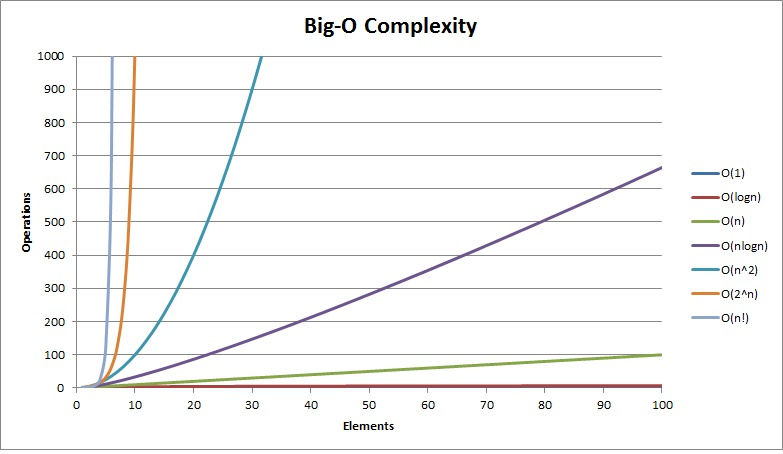# Polynomial Time Algorithms and Intractable Problems

• Which of these are efficient enough? and Which are too inefficient?
• This depends on the problem at hand
• Computer scientists make a distinction
• Distinction between polynomial time algorithms and exponential time algorithms
• Let us say that a function $$f(n)$$ is $$O(g(n))$$ whenever there exists a constant $$c$$ such that $$|f(n)| \leq c \cdot |g(n)|$$ for all values of $$n \geq 0$$

# Polynomial Time Algorithms and Intractable Problems

• Polynomial time algorithm
• One whose time complexity function is $$O(p(n))$$ for some polynomial function $$p$$, where $$n$$ is used to denote the input length
• Exponential time algorithm
• Any algorithm whose time complexity function cannot be so bounded (including functions such as $$n^{log n}$$ which are not normally considered exponential functions)

# Polynomial Time Algorithms and Intractable Problems

• This distinction is important
• When considering the solution to large problem instances
• Explosive growth rates for the exponential complexity functions

# Polynomial Time Algorithms and Intractable Problems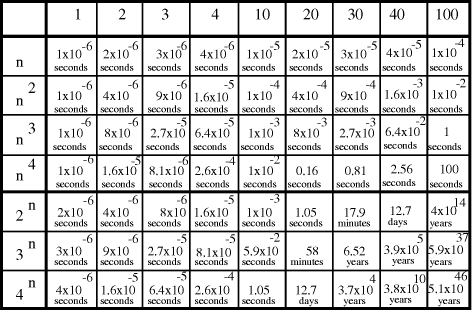# Polynomial Time Algorithms and Intractable Problems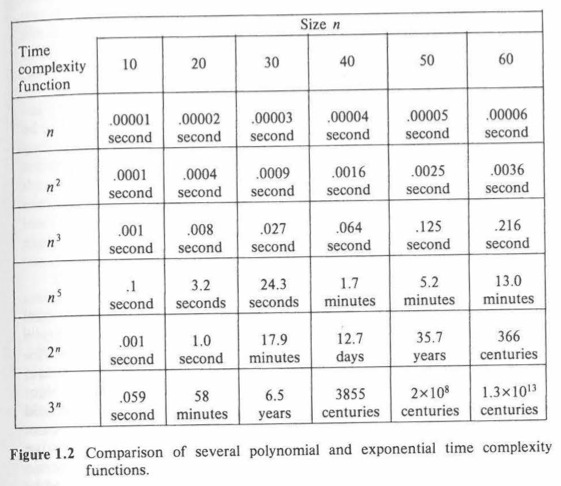# Polynomial Time Algorithms and Intractable Problems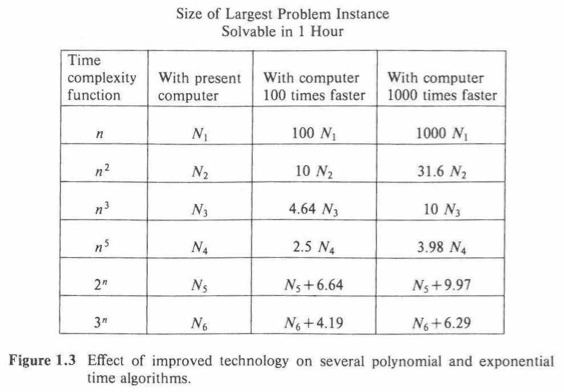# Polynomial Time Algorithms and Intractable Problems

• These tables show why polynomial time algorithms are much more desirable than exponential time ones
• Theory of NP-completeness based on
• Two types of algorithms
• Polynomial time algorithms are much more desirable

# Polynomial Time Algorithms and Intractable Problems

• Exponential time algorithms
• Should not be considered good algorithms
• Most exponential time algorithms are variations on exhaustive search
• Polynomial time algorithms
• Made possible through gain of deeper insight into the structure of the problem
• Agreement that a problem has not been well solved until a polynomial time algorithm is known for it

# Polynomial Time Algorithms and Intractable Problems

• Intractable
• A problem is intractable if it is so hard that no polynomial time algorithm can possibly solve it
• Distinction between efficient polynomial time algorithms and inefficient exponential time algorithms
• Exceptions when problem instances of interest have limited size
• i.e. $$2^n$$ algorithm is faster than the $$n^5$$ algorithm for $$n \leq 20$$ (fig. 1.2)

# Polynomial Time Algorithms and Intractable Problems

• Many exponential time algorithms are useful in practice
• Time complexity defined as a worst-case measure
• Algorithm with time complexity $$2^m$$ means
• At least one instance of size $$n$$ requires that time
• Most problem instances may require less time
• Simplex algorithm (linear programming) shown to have exponential complexity, in practice runs quickly
• Branch and bound algorithms for the knapsack problem are successful

# Polynomial Time Algorithms and Intractable Problems

• Many exponential time algorithms are useful in practice $$\dots$$
• Examples like these are rare
• Many algorithms are known to be exponential but few of them considered as useful in practice

# Polynomial Time Algorithms and Intractable Problems

• Probably efficient algorithms
• Those with degree 2 or 3 at worst
• Do not involve extremely large coefficients
• A desired property

# Polynomial Time Algorithms and Intractable Problems

• Intractability of a problem
• Independent of the encoding scheme and computer model used to obtain time complexity
• Encoding schemes seem to differ at most polynomially from one another
• Reasonable encoding scheme would not differ more than polynomially
• Reasonable, not a formal definition

# Polynomial Time Algorithms and Intractable Problems

• Reasonable encoding
• the encoding of an instance $$I$$ should be concise and not “padded” with unnecessary information or symbols
• numbers occurring in $$I$$ should be represented in binary (or decimal, or octal, or in any fixed base other than 1)
• Follow encodings with these conditions
• Encoding scheme should not affect determination of whether a given problem is intractable

# Polynomial Time Algorithms and Intractable Problems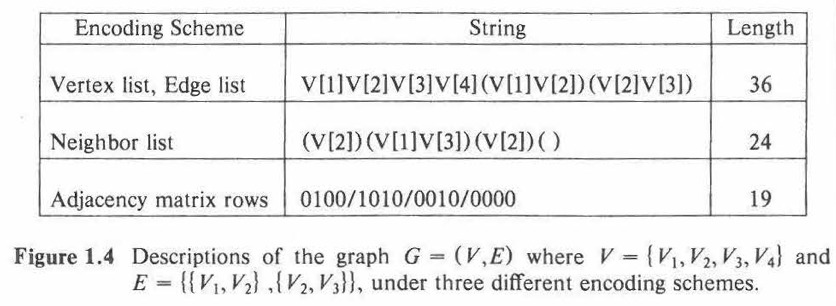# Polynomial Time Algorithms and Intractable Problems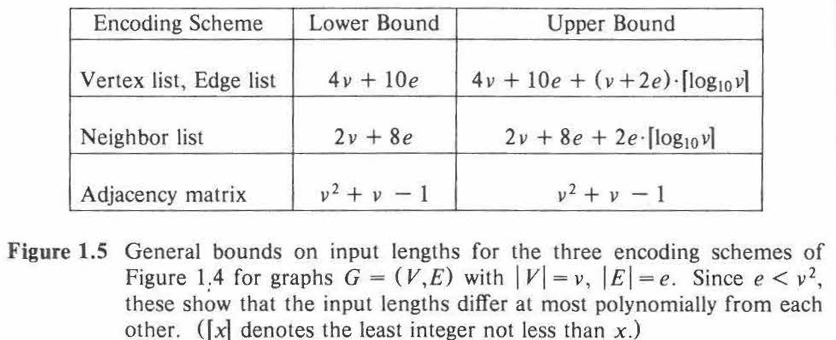# Polynomial Time Algorithms and Intractable Problems

• Similar guidelines for the choice of computer models
• One-tape Turing machines, multi-tape Turing machines, random-access machines (RAMs) $$\rightarrow$$ equivalent
• Reasonable: there is a polynomial bound on the amount of work that can be done in a single unit of time
• Performing arbitrarily many operations in parallel is not considered reasonable
• Not considered for now

# Polynomial Time Algorithms and Intractable Problems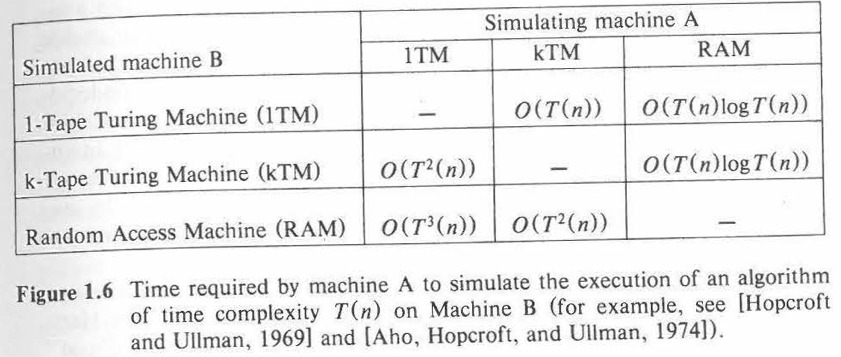# Probably Intractable Problems

• Two causes of intractability allowed by our definition
1. So difficult problem that an exponential amount of time is required to solve it
2. The solution is so extensive that it cannot be described with an expression having length bounded by a polynomial function (the input length)
• i.e. add to TSP parameter B and ask for all tours having total length B or less
• Listing all solutions cannot be done in polynomial time
• This type of complexity is apparent from the problem definition
• The problem is not defined realistically
• We restrict attention to the first type of intractability

# Probably Intractable Problems

• Earliest intractability results
• Decidability results of Alan Turing
• Turing demonstrated that certain problems are so hard that they are undecidable

# Probably Intractable Problems

• Undecidable problems
• No algorithm can be given to solve the problem
• It is impossible to specify any algorithm which
• Given an arbitrary computer program
• Given an arbitrary input to the program
• Can decide whether or not the program will eventually halt when applied to that input
• These problems cannnot be solved by any algorithm
• Much less in $$p$$ time
• They are intractable in a especially strong sense

# Probably Intractable Problems

• Intractable decidable problems
• First ones obtained in the early 1960’s
• Work on complexity hierarchies (Hartmanis and Stearns, 1965)
• Only artificially constructed problems
• In the early 1970’s
• Work by (Meyer and Stockmeyer, 1972), (Fisher and Rabin, 1974),
• Proved some natural decidable problems to be intractable
• Proofs show these problems cannot be solved in polynomial time using even a nondeterministic computer model (computing in parallel)

# Probably Intractable Problems

• Probably intractable problems fall into two categories
• Undecidable
• Non-deterministically intractable
• Most of the apparently intractable problems in practice
• Are decidable
• Can be solved in polynomial time with aid of nondeterministic computer

# NP-Complete Problems

• Study how problems are related with respect to their difficulty
• These relations provide useful information to algorithm designers
• Reducing
• Technique used to demonstrate that two problems are related one to the other
• Transformation that maps any instance of the first problem into an equivalent instance of the second
• Convert any algorithm that solves the second problem into an algorithm for sonving the first one

# NP-Complete Problems

• Foundations of NP-completeness
• The Complexity of Theorem Proving Procedures, Stephen Cook, 1971
• Important things of this paper
• Emphasized significance of polynomial time reducibility
• Transformation (reduction) can be executed by a polynomial time algorithm
• polynomial time reduction from one problem to the other implies that any polynomial time algorithm for the second problem can be converted into a corresponding polynomial time algorithm for the first problem

# NP-Complete Problems

• Foundations of NP-completeness
• The Complexity of Theorem Proving Procedures, Stephen Cook, 1971
• Important things of this paper
• Focused on the class NP of decision problems solvable in polynomial time by a nondeterministic computer
• Decidable problem: its solution is either yes or no
• Most apparently intractable problems (encountered in practice), when described as decision problems, belong to this class

# NP-Complete Problems

• Foundations of NP-completeness
• The Complexity of Theorem Proving Procedures, Stephen Cook, 1971
• Important things of this paper
• Proved that the satisfiability problem has the property: every other problem in NP can be polynomially reduced to it
• If satisfiability problem can be solved with a $$p$$ time algorithm $$\rightarrow$$ every other problem in $$NP$$ can be polynomially reduced to it
• If any problem in $$NP$$ is intractable $$\rightarrow$$ satisfiability problem must also be intractable
• In a sense, satisfiability problem is the hardest problem in $$NP$$

# NP-Complete Problems

• Foundations of NP-completeness
• The Complexity of Theorem Proving Procedures, Stephen Cook, 1971
• Important things of this paper
• Cook suggested other problems in $$NP$$ might be as hard as the satisfiability problem and be members of the $$NP$$ class
• i.e. Does a given graph $$G$$ contain a complete subgraph on a given number of $$k$$ of vertices?
• (Richard Karp, 1972) proved that decision versions of other problems are just as hard as the satisfiability problem (i.e. TSP)

# NP-Complete Problems

• The hardest problems in $$NP$$ are known as the class of NP-complete problems
• There is not a proof that NP-completeness implies intractability
• knowing that a problem is NP-complete suggests that a disruptive algorithm is required to solve it in polynomial time

# Decision Problems, Languages, and Encoding Schemes

• NP-Completeness theory
• Designed to apply only to decision problems (DP)
• DPs have only two possible solutions: yes or no
• Definition
• “A Decision Problem $$\Pi$$ consists of a set $$D_n$$ of instances and a subset $$Y_n \subseteq D_n$$ of yes-instances
• Most problems have more structure than a DP
• We describe them so that they emphasize this structure

# Decision Problems, Languages, and Encoding Schemes

• We use a standard format to specify problems
1. Generic instance of the problem using sets, graphs, functions, numbers, etc.
2. A yes-no question asked in terms of the generic instance
• An instance belongs to $$D_{\Pi}$$ iff it can be obtained from the generic instance by substituting objects of the specified types for all generic components
• An instance belongs to $$Y_{\Pi}$$ iff the answer for the question (specific to an instance) is yes

# Example of Well Known Decision Problems

• Subgraph Isomorphism
• INSTANCE: Two graphs, $$G_1 = (V_1, E_1)$$ and $$G_2 = (V_2, E_2)$$.
• QUESTION: Does $$G_1$$ contain a subgraph isomorphic to $$G_2$$, that is, a subset $$V' \subseteq V_1$$ and a subset $$E' \subseteq E_1$$ such that $$|V'| = |V_2|, |E'| = |E_2|$$, and there exists a one-to-one function $$f:V_2 \rightarrow V'$$ satisfying $$\{u, v\} \in E_2$$ if and only if $$\{f(u), f(v)\} \in E'$$?

# Example of Well Known Decision Problems

• Traveling Salesman
• INSTANCE: A finite set $$C = \{c_1, c_2, \dots, c_m\}$$ of “cities”, a “distance” $$d(c_i, c_j) \in Z^+$$ for each pair of cities $$c_i, c_j \in C$$, and a bound $$B \in Z^+$$ (where $$Z^+$$ denotes the positive integers).
• QUESTION: Is there a “tour” of all the cities in $$C$$ having total length no more than $$B$$, that is, an ordering $$\langle c_{\pi(1)}, c_{\pi(2)}, \dots, c_{\pi(m)} \rangle$$ of $$C$$ such that
$$\Biggl[\sum\limits_{i=1}^{m-1} d(c_{\pi(i)}, c_{\pi(i+1)}) \Biggr] + d(c_{\pi(m)}, c_{\pi(1)}) \leq B$$ ?

# Formal Language

• Set of symbols $$\Sigma$$
• $$\Sigma^*$$, set of finite strings from $$\Sigma$$
• Language over the alphabet $$\Sigma$$
• $$\{01, 10, 001, 110, 0001, 1110\}$$ is a language over $$\{0, 1\}$$
• Correspondence between decision problems and languages
• Related to the encoding schemes to specify problem instances
• Encoding schema $$e$$ for problem $$\Pi$$ used to describe instances of $$\Pi$$ with strings that belong to a fixed alphabet $$\Sigma$$

# Formal Language Setting

• $$\Pi$$ and $$e$$ partition $$\Sigma^*$$ in three classes of strings
1. Strings that are not encodings of instances of $$\Pi$$
2. Strings that encode instances of $$\Pi$$ with answer no
3. Strings that encode instances of $$\Pi$$ with answer yes
• The $$3^{rd}$$ class of strings is the language associated to $$\Pi$$ and $$e$$

• $$L[\Pi, e] = \{x \in \Sigma^* \}$$
• $$\Sigma$$ is the alphabet used by $$e$$, and $$x$$ is the encoding under e of an instance $$I \in Y_{\Pi}$$

# Formal Language Setting

• We apply our language theory to decision problems
• If a result holds for language $$L[\Pi, e]$$, it also holds for the problem $$\Pi$$ under the encoding scheme $$e$$

# Deterministic Turing Machines and the Class P

• We use TMs to formalize the notion of algorithm
• Deterministic one-tape Turing machine (DTM)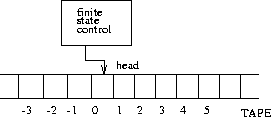# Deterministic Turing Machines and the Class P

• A program in a DTM is specifies as:
• A finite set of symbols $$\Gamma$$, including input symbols $$\Sigma \subset \Gamma$$ and the blank symbol $$b \in \Gamma - \Sigma$$
• A finite set $$Q$$ of states, including a distinguished start state $$q_0$$ and two distinguished halt-states $$q_Y$$ and $$q_N$$
• A transition function $$\delta:(Q-\{q_Y, q_N\}) \times \Gamma \rightarrow Q \times \Gamma \times \{-1, +1\}$$

# Deterministic Turing Machines and the Class P - Example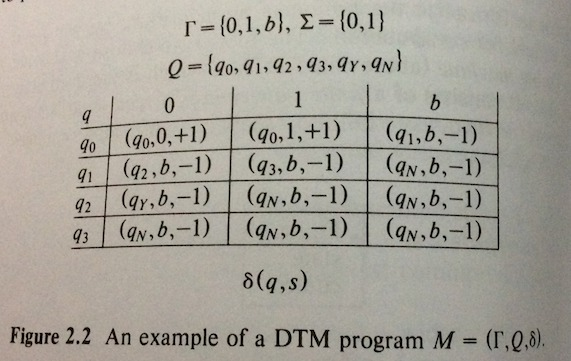-Image from (Garey and Johnson, 1979)

# Deterministic Turing Machines and the Class P - Example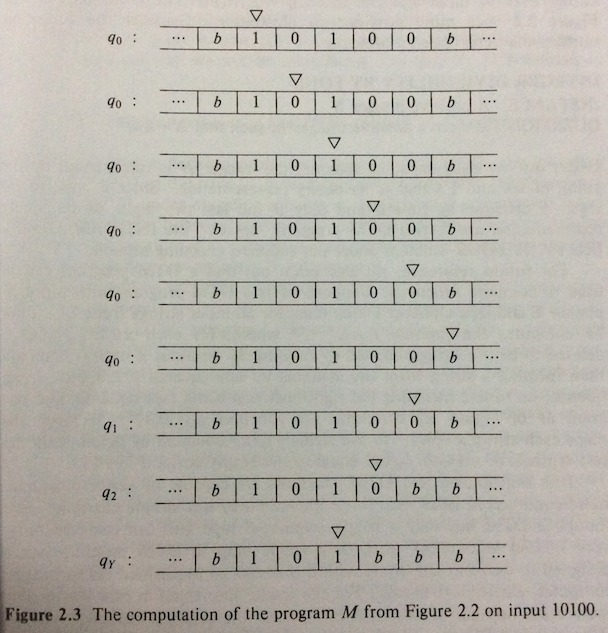-Image from (Garey and Johnson, 1979)

# Deterministic Turing Machines and the Class P - Example

• Computation finishes after 8 steps in state $$q_Y$$
• Answer for instance “10100” is yes

# Deterministic Turing Machines and the Class P

• A DTM program $$M$$ with input alphabet $$\Sigma$$ accepts $$x \in \Sigma^*$$ iff $$M$$ halts in state $$q_Y$$ when applied to input $$x$$
• The language $$L_M$$ recognized by the program $$M$$ is given by
• $$L_M = \{x \in \Sigma^*: M \text{ accepts } x\}$$
• The DTM recognizes the language
• $$\lbrace x \in \{0, 1\}^* : \text{ the rightmost two symbols of x are both 0 }\rbrace$$

# Deterministic Turing Machines and the Class P

• For a DTM program to be an algorithm, it must halt on all possible strings over its input alphabet
• DTM program of Fig. 22.2 is algorithmic, it halts for any input string from $$\{0, 1\}^*$$

# Deterministic Turing Machines and the Class P

• Correspondence between recognizing languages and solving decision problems
• A DTM program $$M$$ solves the decision problem $$\Pi$$ under encoding scheme $$e$$ if $$M$$ halts for all input strings over its input alphabet and $$L_M = L[\Pi, e]$$

# Deterministic Turing Machines and the Class P

• Formal definition of time complexity
• The time used in the computation of a DTM program $$M$$ on an input $$x$$ is the number of steps occurring on that computation up until a halt state is entered
• For a DTM program $$M$$ that halts for all inputs $$x \in \Sigma^*$$, its time complexity function $$T_M(n) : Z^+ \rightarrow Z^+$$ is given by

\begin{aligned} T_M(n) = {} & \text{max} \lbrace m : \mbox{there is an } x \in \Sigma^* \mbox{, } \mbox{with } |x|=n \mbox{, such that}\\ & \mbox{the computation of } M \mbox{ on input } x \mbox{ takes time } m \rbrace \end{aligned}

# Deterministic Turing Machines and the Class P

• Program $$M$$ is called a polynomial time DTM program if there exists a polynomial $$p$$ such that, for all $$n \in Z^+$$, $$T_M(n) \leq p(n)$$

# The P Class

\begin{aligned} P = {} & \lbrace L : \mbox{there is a polynomial time DTM program } M\\ & \mbox{ for which } L = L_M \rbrace \end{aligned}

• A decision problem $$\Pi$$ belongs to $$P$$ under encoding scheme $$e$$ if $$L[\Pi, e] \in P$$
• Language $$L$$ represents instances of $$\Pi$$ with encoding $$e$$
• $$L$$ is equivalent to the language of program $$M$$ ($$L_M$$)
• Polynomial time DTM program that solves $$\Pi$$ under $$e$$
• We say that decision problem $$\Pi$$ belongs to $$P$$
• $$M$$ always gives the right answer for the problem at hand

# Non Deterministic Computation and the Class NP

• Suppose we have a problem that we know there is not a polynomial time algorithm to solve it
• The Traveling Salesman problem
• Subgraph Isomorphism problem
• Somebody claims that he has found a solution
• We want to prove such a claim is true, VERIFY A yes ANSWER for that particular instance of the problem
• Prove the required properties (as in the problem description)

# Non Deterministic Computation and the Class NP

• Verification procedure $$\rightarrow$$ a general algorithm with time complexity polynomial in Length[$$I$$]
• Polynomial time verifiability
• DOES NOT IMPLY POLYNOMIAL TIME SOLVABILITY

# Non Deterministic Computation and the Class NP

• Define NP in terms of nondeterministic algorithm, given problem instance $$I$$
• Guessing stage: guesses some structure $$S$$
• Checking stage: takes $$I$$ and $$S$$ as inputs
• Compute in normal deterministic way, halting in yes, or no, or computing forever

# Non Deterministic Computation and the Class NP

• Nondeterministic algorithm solves a decision problem $$\Pi$$ if two properties hold for all instances $$I \in D_{\Pi}$$
• If $$I \in Y_{\Pi}$$
• There exists structure $$S$$ that, when guessed for input $$I$$, will lead the checking stage to respond yes for $$I$$ and $$S$$
• If $$I \notin Y_{\Pi}$$
• There exists no structure $$S$$ that, when guessed for input $$I$$, will lead the checking stage to respond yes for $$I$$ and $$S$$

# Non Deterministic Computation and the Class NP

• Nondeterministic algorithm solves decision problem $$\Pi$$ in polynomial time if:
• There exists polynomial $$p$$ such that foreach instance $$I \in Y_{\Pi}$$, there is some guess $$S$$ that leads the deterministic checking stage to respond yes for $$I$$ and $$S$$ within time $$p(Length[I])$$

# Non Deterministic Computation and the Class NP

• Informally, class NP is defined as
• The class of all decision problems $$\Pi$$ that, under reasonable encoding schemes, can be solved by polynomial time nondeterministic algorithms
• Polynomial time nondeterministic algorithm
• Our device for polynomial time verifiability
• Not a realistic method to solve decision problems
• For a given input, it has many possible computations, one for each possible guess

# Non Deterministic Computation and the Class NP

• Formal counterpart of a nondeterministic algorithm
• Program for a nondeterministic one-tape Turing Machine (NDTM)
• We augment our DTM with a guessing module with its own write-only head
• Writes the guess to the tape